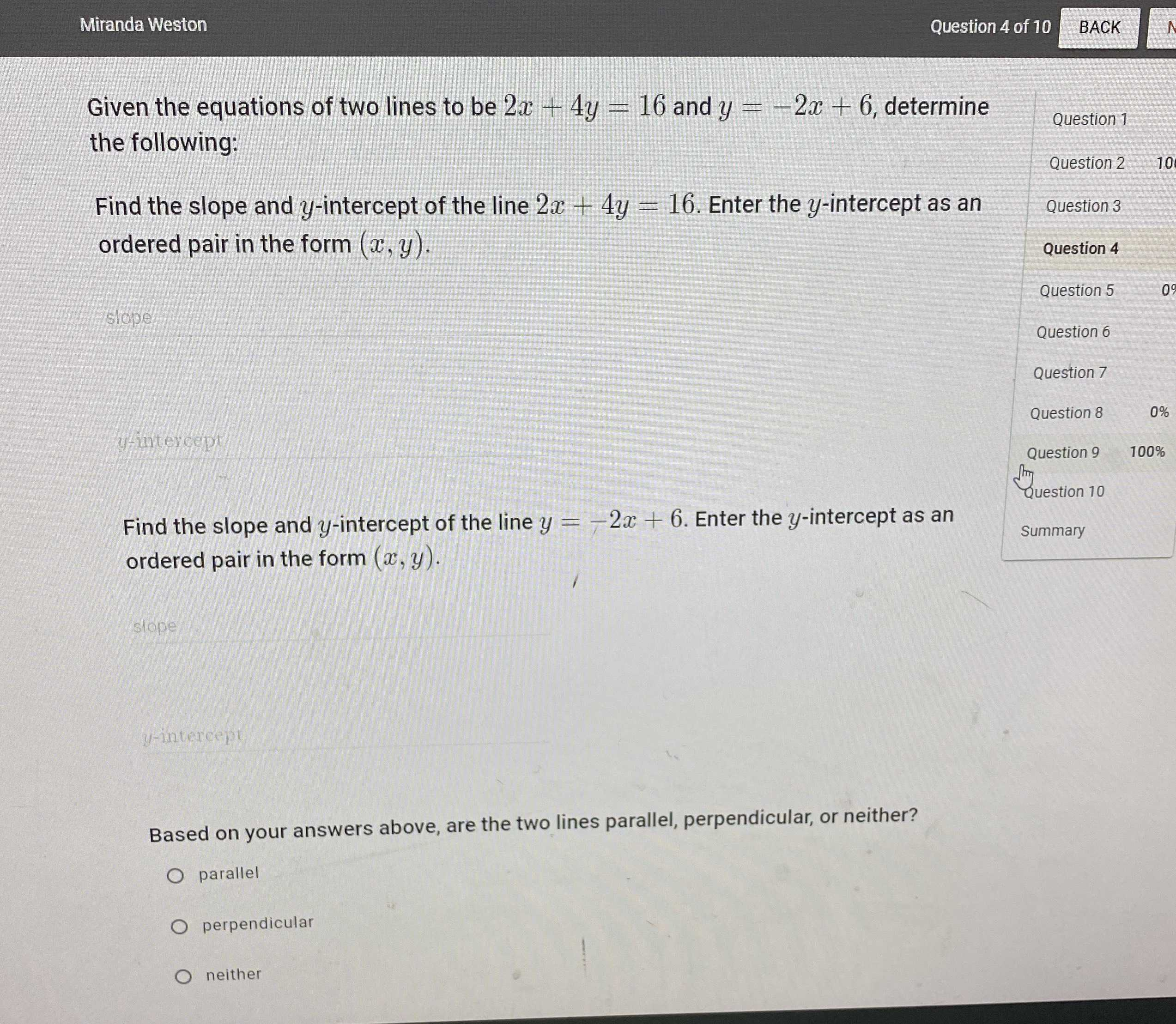### Still have math questions?

Algebra
QuestionGiven the equations of two lines to be $$2 x + 4 y = 16$$ and $$y = - 2 x + 6 ,$$ determine the following:

Find the slope and $$y$$ -intercept of the line $$2 x + 4 y = 16$$ . Enter the $$y$$ -intercept as an ordered pair in the form $$( x , y )$$ .

Find the slope and $$y$$ -intercept of the line $$y = - 2 x + 6$$ . Enter the $$y$$ -intercept as an ordered pair in the form $$( x , y )$$ .

Based on your answers above, are the two lines parallel, perpendicular, or neither?

line 2x+4y=16

slope=$$- \frac{1}{2}$$

y-intercept: (0, 4)

line y=-2x+6

slope=-2

y-intercept: (0, 6)

Based on the answers above, the two lines are neither

Solution
View full explanation on CameraMath App.